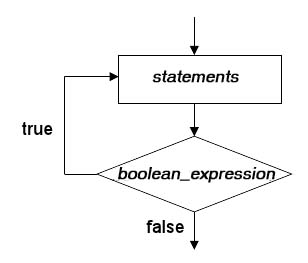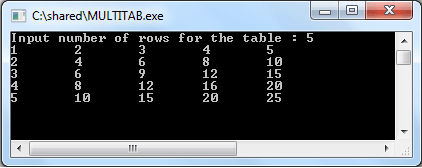# C do while loop

## Description

do...while loops are almost same like while loops, except the condition, is checked at the end of the loop, instead of at the beginning. Therefore the code in the do-while loop will always be executed at least once. The general form is shown below :

do

{

statement(s);

}while (condition)

The sequence of operations is as follows :

1. execute the code within the braces

2. check the condition (boolean expression) and if it is true, go to step 1 and repeat

3. this repetition continues until the condition (boolean expression) evaluates to false.

See the flowchart :## Nested do while loop

The following program will print out a multiplication table of numbers 1,2,…,n. The outer do-while loop is the loop responsible for iterating over the rows of the multiplication table. The inner loop will, for each of the values of colnm, print the row corresponding to the colnm multiplied with rownm. Here we use the special "\t" character within printf() function to get a clear output.

``````#include <stdio.h>
main()
{
int rownm,nrow,colnm;
rownm=1;
colnm=1;
printf("Input number of rows for the table : ");
scanf("%d",&nrow);
do
{
colnm=1;
do
{
printf("%d\t",rownm*colnm);
colnm++;
}
while(colnm<=nrow);
rownm++;
printf("\n");
}
while(rownm<=nrow);
}
``````

Output:Previous: C while loop
Next: C array

﻿

## C Programming: Tips of the Day

What does [ N ... M ] mean in C aggregate initializers?

It is initialization using Designated Initializers.

The range based initialization is a gnu gcc extension.

To initialize a range of elements to the same value, write [first ... last] = value. This is a GNU extension. For example,

``` int widths[] = { [0 ... 9] = 1, [10 ... 99] = 2,  = 3 };
```

It is not portable. Compiling with -pedantic with tell you so.

How does it work here?

The preprocessor replaces #include <asm/unistd.h> with its actual contents(it defines miscellaneous symbolic constants and types, and declares miscellaneous functions) in the range based construct, which are then further used for initializing the array of pointers.

Ref : https://bit.ly/2SQE4wm